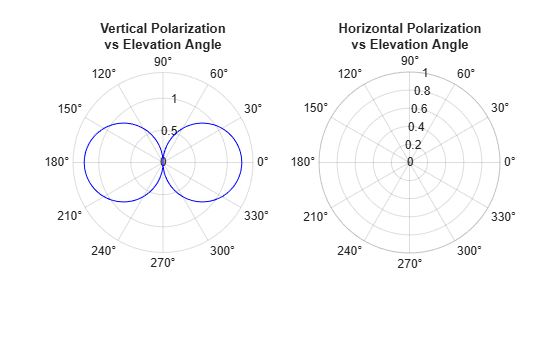## Short-dipole Antenna Element

When you want to explicitly study the effects of polarization in a radar or communication system, you need to specify an antenna that can generate polarized radiation. One such antenna is the short-dipole antenna, created by using the `phased.ShortDipoleAntennaElement`.

The simplest polarized antenna is the dipole antenna which consist of a split length of wire coupled at the middle to a coaxial cable. The simplest dipole, from a mathematical perspective, is the Hertzian dipole, in which the length of wire is much shorter than a wavelength. A diagram of the short dipole antenna of length L appears in the next figure. This antenna is fed by a coaxial feed which splits into two equal length wires of length L/2. The current, I, moves along the z-axis and is assumed to be the same at all points in the wire.The electric field in the far field has the form

`$\begin{array}{l}{E}_{r}=0\\ {E}_{H}=0\\ {E}_{V}=-\frac{i{Z}_{0}IL}{2\lambda }\mathrm{cos}\text{el}\text{ }\frac{{e}^{-ikr}}{r}\end{array}$`

The next example computes the vertical and horizontal polarization components of the field. The vertical component is a function of elevation angle and is axially symmetric. The horizontal component vanishes everywhere.

### Short-Dipole Polarization Components

Compute the vertical and horizontal polarization components of the field created by a short-dipole antenna pointed along the z-direction. Plot the components as a function of elevation angle from 0° to 360°.

Create the `phased.ShortDipoleAntennaElement` System object™.

```antenna = phased.ShortDipoleAntennaElement(... 'FrequencyRange',[1,2]*1e9,'AxisDirection','Z');```

Compute the antenna response. Because the elevation angle argument to `antenna` is restricted to ±90°, compute the responses for 0° azimuth and then for 180° azimuth. Combine the two responses in the plot. The operating frequency of the antenna is 1.5 GHz.

```el = -90:90; az = zeros(size(el)); fc = 1.5e9; resp = antenna(fc,[az;el]); az = 180.0*ones(size(el)); resp1 = antenna(fc,[az;el]);```

Overlay the responses in the same figure.

```figure(1) subplot(121) polarplot(el*pi/180.0,abs(resp.V.'),'b') hold on polarplot((el+180)*pi/180.0,abs(resp1.V.'),'b') str = sprintf('%s\n%s','Vertical Polarization','vs Elevation Angle'); title(str) hold off subplot(122) polarplot(el*pi/180.0,abs(resp.H.'),'b') hold on polarplot((el+180)*pi/180.0,abs(resp1.H.'),'b') str = sprintf('%s\n%s','Horizontal Polarization','vs Elevation Angle'); title(str) hold off```The plot shows that the horizontal component vanishes, as expected.